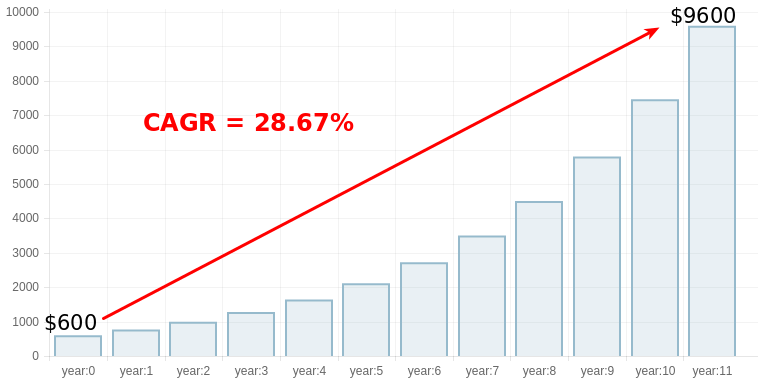# What Is The Formula For Calculating CAGR (Compound Annual Growth Rate)

The CAGR or compound annual growth rate is the average rate at which an investment grows over time assuming that it was compounded (re-invested) annually (periodically).

CAGR has nothing to do with the value of an investment in the intermediate years as it depends only upon the value in the first year and the last year of the investment tenure.

Also see : Online CAGR Calculator

## CAGR Formula :The formula for CAGR is:

CAGR = ( FV / SV )1 / N - 1

where:

FV = final value of an investment
SV = starting value of an investment
N = total number of investment periods (months, years, etc.)

## Applying CAGR Formula with an example

Let's see how to use the above formula. Suppose we have following data for years and the investment value in the respective year.

 YEAR AMOUNT 2008 \$1000 2009 \$1342 2010 \$1255 2011 \$1468 2012 \$1699 2013 \$1927 2014 \$2466 2015 \$2134 2016 \$2507

As you can see in the above table the investement starts from year 2008and ends in year 2016. So, here the total number of period for which we want to calculate CAGR is 9 years ( year 2008 is also taken into account). Next, in the table the value for the year 2008 and 2016 is \$1000 and \$2507 respectively. Applying these known values into the above mentioned formula, we have:

N = 9; SV = 1000; FV = 2507

The expression would be :

CAGR = ( 2507 / 1000 )1 / 9 - 1 = 0.1075

CAGR (%) = 0.1075 x 100 = 10.75 %

## Another Example with CAGR Formula

Let's understand with another example. If you had purchased 10 stocks of Amazon.com Inc (NASDAQ) at \$60 each share in May 2007, the starting value (SV) would be \$600. Today (as on May 2017 ) the final value (FV) of your investment should be \$9600 with each share trading at \$960.The total year that it took to grow is 11 years. So, in this case the known values are :

N = 11; SV = 600; FV = 9600

The expression for calculation would be :

CAGR = ( 9600 / 600 )1 / 11 - 1 = 0.2867

CAGR (%) = 0.2867 x 100 = 28.67 %

Your investment in Amazon.com Inc stocks would had grown at CAGR of 28.67